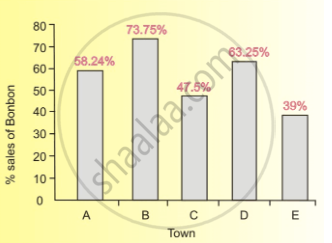# If the Total Consumption of Modern Bread in Town B is 2100 Loaves, Then Find the Total Consumption of Bread in All the Five Cities. - Mathematics

MCQ

Direction:  Answer the question on the basis of the information given below.

The following information is about the consumption of bread in five towns - A, B, C, D and E. Town A consumes a quarter of the total quantity of bread sold in all the five cities. The bread consumption of Town B is one-fourth of the consumption of Town C. The bread consumption of Town D is 12% of the total whereas Town E consumes 23% of the total.

There are only two companies - Bonbon and Modern - that sell bread in these five towns. The bar graph shows the sales of Bon-bon as a percentage of the total sale of bread in each city.If the total consumption of Modern bread in Town B is 2100 loaves, then find the total consumption of bread in all the five cities.

• 90000

• 120000

• 50000

• 100000

#### Solution

100000

Explanation:

According to the information given, The consumption of Modern bread in Towns B is 26.25% of 8% of the total consumption, which is given as 2100 loaves. Hence, the total consumption can be calculated as :

(2100/26.25 xx 100) xx 100/8 = 100000 loaves

Concept: Graph and Charts (Entrance Exam)
Is there an error in this question or solution?